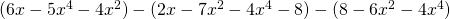Chapter 6: Polynomials

# 6.4 Basic Operations Using Polynomials

Many applications in mathematics have to do with what are called polynomials. Polynomials are made up of terms. Terms are a product of numbers and/or variables. For example,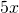,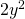,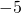,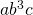, and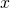are all terms. Terms are connected to each other by addition or subtraction.

Expressions are often defined by the number of terms they have.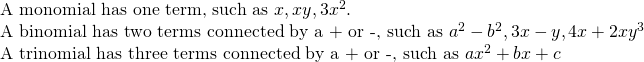The term polynomial is generic for many terms. Monomials, binomials, trinomials, and expressions with more terms all fall under the umbrella of “polynomials.”

Polynomials are classified by the sum of exponents of the term with the highest exponent sum, which is called the degree of the polynomial.

A first degree polynomial is a linear polynomial and would not have any terms with a sum of exponents greater than one. Examples include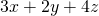,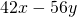,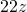.

A second degree polynomial is a quadratic polynomial and would not have any terms with a sum of exponents greater than two. Examples include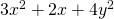,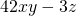,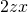.

A third degree polynomial is a cubic polynomial and would not have any terms with a sum of exponents greater than three. Examples include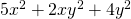,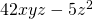,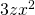.

A fourth degree polynomial is a quartic polynomial and would not have any terms with a sum of exponents greater than four. Examples include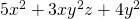,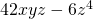,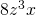.

A fifth degree polynomial is a quintic polynomial.

A sixth degree polynomial is a sextic polynomial.

A seventh degree polynomial is a septic polynomial.

A eighth degree polynomial is a octic polynomial.

A ninth degree polynomial is a nonic polynomial.

A tenth degree polynomial is a decic polynomial.

The degree of any term is the sum of its exponents: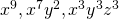are all ninth degree terms (nonic polynomial)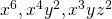are all sixth degree terms (sextic polynomial)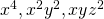are all fourth degree terms (quartic polynomial)

Terms of a polynomial are named in the order of their appearance. For instance, the polynomial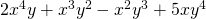has four terms, each one of the fifth degree. The terms are numbered in order for this polynomial, starting from the first term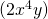and continuing to the second ((x^3y^2)\), third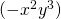, and fourth terms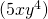.

If it is known what the variable in a polynomial represents, it is possible to substitute in the value and evaluate the polynomial, as shown in the following example.

Example 6.4.1

Simplify the expression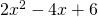using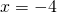.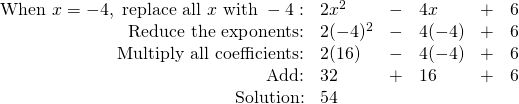Remember the exponent only affects the number to which it is physically attached. This means −32 = −9 because the exponent is only attached to the 3, whereas (−3)2 = 9 because the exponent is attached to the parentheses and everything inside.

Example 6.4.2

Simplify the expression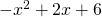using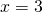.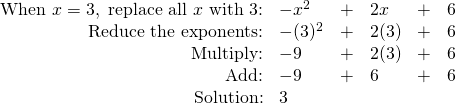Sometimes, when working with polynomials,  the value of the variable is unknown and the polynomial will be simplified rather than ending with some value. The simplest operation with polynomials is addition. When adding polynomials, it is merely combining like terms. Consider the following example.

Example 6.4.3

Simplify the expression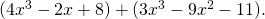The first thing one should do is place the equations one over top of the other, ordering each of the terms into columns so they can simply be added or subtracted.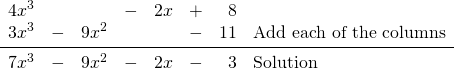Subtracting polynomials is almost as fast as addition. Subtraction is generally shown by a minus sign in front of the parentheses. When there is a negative sign in front of parentheses,  distribute it throughout the expression, changing the signs of everything inside.

Example 6.4.4

Simplify the expression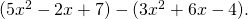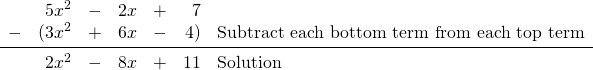Example 6.4.5

Simplify the expression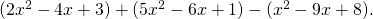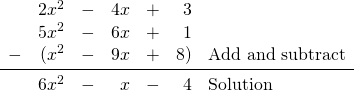# Questions

For questions 1 to 8, simplify each expression using the variables given.

1.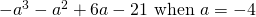2.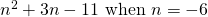3.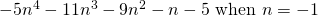4.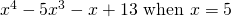5.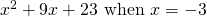6.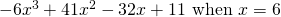7.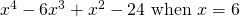8.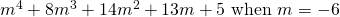For questions 9 to 28, simplify the following expressions.

1.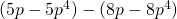2.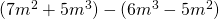3.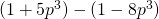4.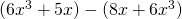5.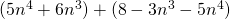6.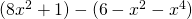7.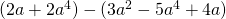8.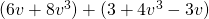9.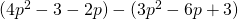10.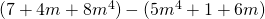11.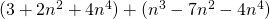12.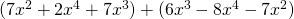13.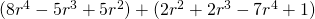14.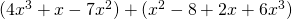15.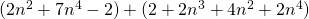16.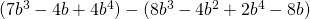17.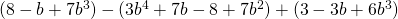18.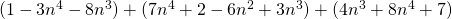19.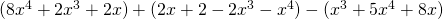20.